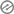# An easy mathematics quiz for kindergarten aims to introduce basic math concepts in a fun and interactive way

An easy mathematics quiz for kindergarten aims to introduce basic math concepts in a fun and interactive way. Here are some sample questions for such a quiz:

1. How many fingers do you have on one hand?
a) 4
b) 5
c) 6

2. Count the number of apples: [Image with three apples]
a) 2
b) 3
c) 43. Which shape has three sides?
a) Circle
b) Square
c) Triangle

4. Add the following numbers: 2 + 1 =
a) 2
b) 3
c) 4

5. How many legs does a dog have?
a) 2
b) 3
c) 46. What comes after the number 3?
a) 2
b) 3
c) 4

7. Which picture shows five objects? [Image with five balloons]
a)
b)
c)

8. How many sides does a square have?
a) 2
b) 3
c) 49. Subtract the following numbers: 4 – 2 =
a) 2
b) 3
c) 4

10. How many colors are there in a rainbow?
a) 3
b) 5
c) 7

These questions are designed to assess basic counting skills, number recognition, simple addition and subtraction, and shape identification. The quiz can be presented visually with images to make it more engaging for kindergarten students.